# Calculus

Calculus in Maths deals with continuous change. Derivatives and integrals are the two most important parts of calculus. In other words, we can say that calculus is the study of the continuous change of functions. The integral gives us the area under the curve, while the derivative gives us the rate of change of a function. The integral gathers the different values of a function over a number of values, while the derivative describes the function at a particular point.

The Two major concepts of calculus are: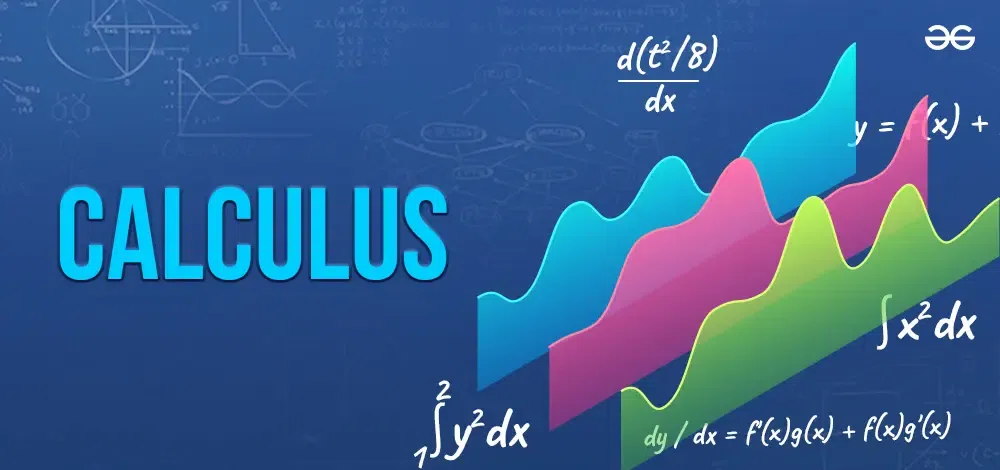## What is Calculus?

Calculus, a branch of mathematics founded by Newton and Leibniz, deals with the pace of transition. Calculus Math is commonly used in mathematical simulations to find the best solutions. It aids us in understanding the changes between values that are linked by a purpose. Calculus Math is mostly concerned with certain critical topics such as separation, convergence, limits, functions, and so on.

## Calculus Definition

Calculus is the part of maths that deals with the properties of derivatives and integrals of quantities like area, volume, velocity, acceleration, etc.

## Basic Calculus

Calculus delves into differentiation and integration, rooted in limits and functions. Fundamental concepts such as continuity and exponents serve as the bedrock for advanced calculus. There are two main types: Differential Calculus, used to determine the rate of change, and Integral Calculus, used to find quantity based on known rates of change.

## Calculus Topics

Depending upon the variety of topics covered under calculus, we can divide the topics into different categories as listed below,

• Precalculus
• Calculus 1
• Calculus 2

### Precalculus

Precalculus is a domain of mathematics that consists of trigonometry and algebra created to get students ready for the preparation of calculus. In precalculus, advanced mathematics is mainly focused upon which includes functions and quantitative reasoning. Major topics studied under precalculus are,

• Functions
• Inverse Numbers
• Complex Numbers
• Rational Function

### Calculus 1

Calculus consists of topics mainly focusing on differential calculus and similar concepts like limits and continuity. Some topics under Calculus 1 are,

• Limits
• Derivatives
• Applications of Derivatives

### Calculus 2

Calculus 2 is all about the mathematical study of change that occurred during the modules of Calculus 1. Some of the topics covered under Calculus 1 are,

• Differential Equations
• Sequence and Series
• Application of Integrals
• Trapezoidal Rule

## Functions

In calculus, functions denote the relationship between two variables, which are independent and the dependent variable.

Let’s examine the following diagram.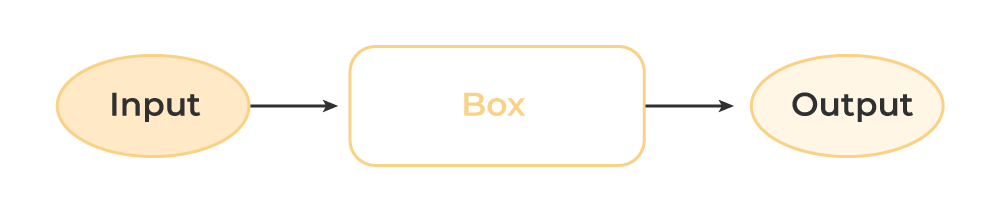We can see that there is an INPUT, a box, and an OUTPUT. For example, assume we want to bake a cake. We would require the following ingredients.

• Refined Flour
• Sugar
• Eggs
• Butter
• Baking powder
• Baking soda

The above example can be represented as a function as shown below,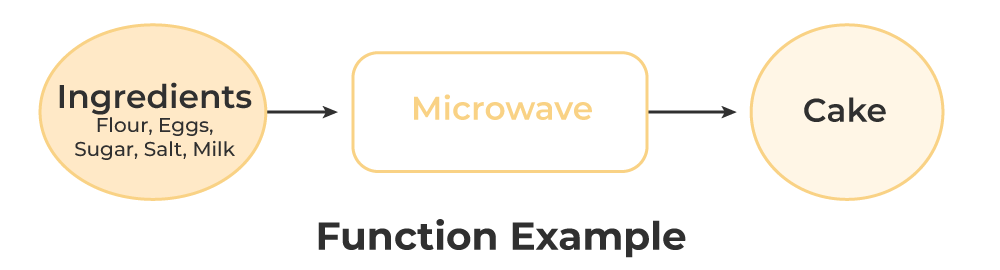Let’s take another example,

y=5x

Value of x Value of y
1 5
2 10
3 15
4 20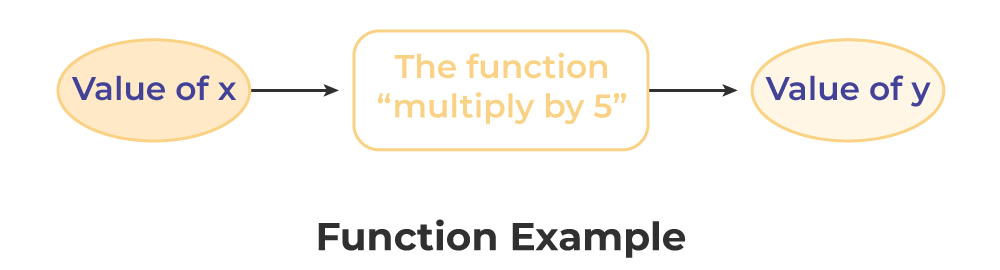From the above example, we can see that the value of y depends on the value of x. We can conclude that

• INPUT is independent of the OUTPUT
• INPUT is independent of the OUTPUT
• OUTPUT depends on the INPUT
• The box is accountable for the change of the INPUT to the OUTPUT

In calculus,

• INPUT is an independent variable
• OUTPUT is a dependent variable
• The box is a function

## Types of Calculus

Calculus Mathematics can be divided into two types: Differential Calculus and Integral Calculus. Both differential and integral calculus consider the effect of a small shift in the independent variable on the equation as it approaches zero. Both discrete and integral calculus serves as a basis for the higher branch of mathematics known as Analysis.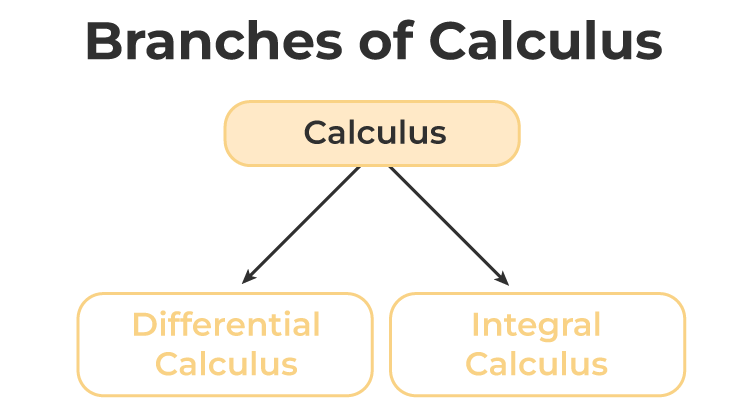## Differential Calculus

Differential calculus is used to solve the problem of calculating the rate at which a function changes in relation to other variables. To obtain the optimal answer, derivatives are utilized to determine a function’s maxima and minima values. It primarily handles variables like x and y, functions like f(x), and the variations in x and y that follow. dy and dx are used to symbolize differentials. The process of differentiating allows us to compute derivatives. The derivative of a function is given by dy/dx or f’ (x). Let’s go over some of the important subjects covered in basic differential calculus.

### Limits

Limit is used to calculate the extent of closeness to any term or upcoming term. A limit is denoted with the help of the limit formula as,

limx⇢cf(x) = A

This expression is understood as “the limit of f of x approaches c equals A”.

## Derivatives

The instantaneous rate at which one quantity changes in relation to another is represented by derivatives. The representation of a function’s derivative is:

limx⇢h[f(x + h) – f(x)]/h = A

## Continuity

A function f(x) is said to be continuous at a particular point x = a if the following three conditions are satisfied –

• f(a) is defined
• limx⇢af(x) exists
• limx⇢a– f(x) = limx⇢a+ f(x) =f(a)

## Continuity and Differentiability

A function is always continuous if it is differentiable at any point, whereas the vice-versa condition is not always true.

### Integral Calculus

The study of integrals and their properties is known as integral calculus. It is primarily useful for:

• To compute f from f’ (i.e. from its derivative). If a function f is differentiable in the range under consideration, then f’ is specified in that range.
• To determine the region under a curve.

### Integration

Integration is exactly the opposite of differentiation. Differentiation is the partition of a portion into a number of smaller parts, and integration is gathering tiny parts to create a whole. It is frequently applied to area calculations.

### Definite Integral

A definite integral has a specified boundary beyond which the equation must be computed. The lower and upper limits of a function’s independent variable are defined, and its integration is represented using definite integrals.### Indefinite Integral

An infinite integral lacks a fixed boundary, i.e. there is no upper and lower limit. As a result, the integration value is always followed by a constant value.Following are the articles that discuss integral calculus deeply:

## Calculus Formulas

The Calculus formulas used in calculus can be divided into six major categories. The six major formula categories are limits, differentiation, integration, definite integrals, application of differentiation, and differential equations.

### Limits Formulas

Limits Formulas help in estimating the values to a definite number and are defined either to zero or to infinity.

Ltx⇢0(xn – an)(x-a)=na(n-1)

Ltx⇢0(sin x)/x = 1

Ltx⇢0(tan x)/x = 1

Ltx⇢0(ex – 1)/x = 1

Ltx⇢0(ax – 1)/x = logea

Ltx⇢0(1 +(1/x))x = e

Ltx⇢0(1 + x)1/x = e

Ltx⇢0(1 + (a/x))x= ea

### Differentiation Formulas

These formulas can be applied to algebraic expressions, trigonometric ratios, inverse trigonometry, and exponential terms.### Integration Formula

Integrals Formulas can be derived from differentiation formulas, and are complimentary to differentiation formulas.

∫ xn.dx = xn + 1/(n + 1) + C
∫ 1.dx = x + C
∫ ex.dx = ex + C
∫(1/x).dx = log|x| + C
∫ ax.dx = (ax/log a) + C
∫ cos x.dx = sin x + C
∫ sin x.dx = -cos x + C
∫ sec2x.dx = tan x + C
∫ cosec2x.dx = -cot x + C
∫ sec x.tan x.dx = sec x + C
∫ cosec x.cotx.dx = -cosec x + C

### Definite Integrals Formulas

Definite Integrals are the basic integral formulas with limits. There is an upper and lower limit, and definite integrals, that are helpful in finding the area within these limits.f(x).dx=f(t).dt= –f(x).dxcf(x).dx = cf(x).dxf(x) ± g(x).dx =f(x).dx ±g(x).dxf(x).dx =f(x).dx +f(x).dxf(x).dx =f(a+b-x).dxf(x).dx =f(a-x).dxf(x).dx =  2f(x).dx if f(2a-x)=f(x)f(x).dx = 0 if f(2a-x) = -f(x)f(x).dx = 2f(x).dx if f(x) is an even function (i.e., f(-x)= f(x)).f(x).dx = 0 if f(x) is an odd function (i.e., f(-x) = -f(x)).

### Differential Equations formula

Differential equations can be compared to general equations because they are higher-order derivatives. In the general equation, the variable ‘x’ is an unknown, and in this case, the variable is the differentiation of dy/dx.

Homogeneous Differential Equation : f(λx, λy)= λnf(x,y)

Linear Differential Equation: dy/dx +Py = Q
The general solution of the Linear Differential Equation is y.e-∫P.dx = ∫(Q.e∫P.dx ).dx + C

## Applications of Calculus

Calculus plays a very important role and helps us in :

• Examining a system to discover the best approach to forecast any given circumstance for a function.
• Calculus concepts are widely used in everyday life, whether it is to solve problems with complex shapes, assess survey results, determine the safety of automobiles, design a business, track credit card payments, or determine how a system is developing and how it affects us, etc.
• Economists, biologists, architects, doctors, and statisticians all speak calculus. For instance, engineers and architects employ several calculus ideas to determine the size and design of construction structures.
• Modeling ideas like occurrence and mortality rates, radioactive decay, reaction rates, heat and light, motion, and electricity all employ calculus.

Advanced Calculus builds upon basic principles such as differentiation and derivatives, extending into topics like infinite series and power series. Essential areas for advanced study include vector spaces, matrices, and linear transformations. This advanced branch explores intricate concepts like quadratic forms, the Generalized Stokes theorem, integration of forms, multilinear algebra, and critical point analysis for multivariate functions. It also delves into vector fields as derivatives, continuous differentiability, tangent space, normal space via gradients, and the dual space with its dual basis, providing profound insights into complex mathematical relationships.

## Sample Problems on Calculus

Problem 1: Find the percentage error in calculating the surface area of a cubical box if an error of 1% is made in measuring the lengths of edges of the cube.

Solution:

According to the given condition,

Let △x be the error in the length and △y be the error in the surface area

Let’s take length as x

△x/x × 100 = 1

△x = x/100

x+△x = x+(x/100)

As, surface area of the cube = 6x2

dy/dx= 6(2x) = 12x

△y = dy/dx △x

△y = (12x) (x/100)

△y = 0.12 x2

So, △y/y = 0.12 x2/6 x2 = 0.02

Percentage change in y = △y/y × 100 = 0.02 × 100 = 2

Hence, the percentage error in calculating the surface area of a cubical box is 2%

Problem 2: If x3 + y3 = 3axy, find dy/dx.

Solution:

Given, x3 + y3 = 3axy

Differentiating both sides with respect to x, we get:

3x2 + 3y2 (dy/dx) = 3ay + 3ax (dy/dx)

⇒ {3y2 – 3ax} (dy/dx) = 3ay – 3x2

⇒ dy/dx = (3ax – 3x2)/(3y2 – 3ax)

⇒ dy/dx = (ax – x2.)/(y2 – ax).

Also Check:

## FAQs – Calculus

### 1. What is Calculus?

Calculus is a branch of mathematics that studies the rate of change. It’s used in mathematical models to find optimal solutions. Calculus is concerned with two basic operations, differentiation and integration.

### 2. What is Differential Calculus?

Differential calculus is used to study the problems of calculating the rate at which a function changes in relation to other variables. It is represented in the form of

f'(x) = dy/dx

### 3. What is Integral Calculus?

The process of calculating the area under a curve or a function is called integral calculus.

### 4. What is the maxima and minima of a function?

Maxima is the highest value of a function while minima is the lowest value of a function. Both can be obtained by finding the derivative of a function.

### 5. What are the 4 types of calculus?

The four main concepts covered under calculus are given below,

• Limits
• Differential Calculus
• Integral Calculus
• Multivariable Calculus

### 6. What do you Use Calculus for?

Calculus is fundamental to understanding and describing the behavior of physical systems. Engineers use calculus to analyze and design structures, and electrical circuits. Calculus is used to develop financial models and analyze complex financial derivatives.

### 7. What is the Meaning of Calculus?

Calculus, a vital branch of mathematics, studies derivatives and integrals to understand dynamic quantities like area, volume, velocity, and acceleration. It reveals changes within functions and provides essential tools for analyzing complex relationships between variables.

Whether you're preparing for your first job interview or aiming to upskill in this ever-evolving tech landscape, GeeksforGeeks Courses are your key to success. We provide top-quality content at affordable prices, all geared towards accelerating your growth in a time-bound manner. Join the millions we've already empowered, and we're here to do the same for you. Don't miss out - check it out now!

Related Tutorials